# NCERT Solutions for Class 10 Maths Exercise 14.4## myCBSEguide App

CBSE, NCERT, JEE Main, NEET-UG, NDA, Exam Papers, Question Bank, NCERT Solutions, Exemplars, Revision Notes, Free Videos, MCQ Tests & more.

NCERT solutions for Maths Statistics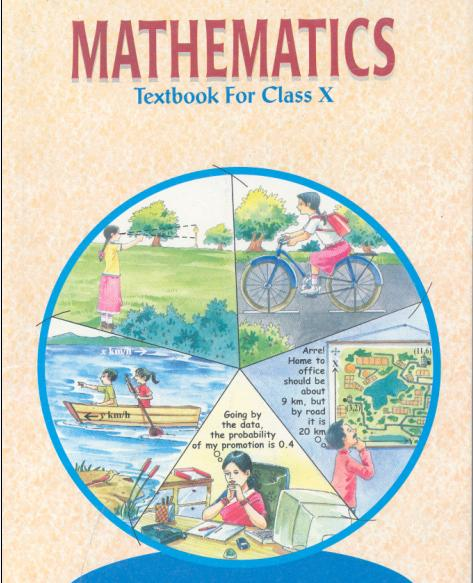## NCERT Solutions for Class 10 Maths Statistics

1. The following distribution gives the daily income of 50 workers of a factory: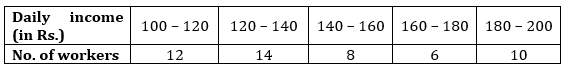Convert the distribution above to a less than type cumulative frequency distribution and draw its ogive.

Ans.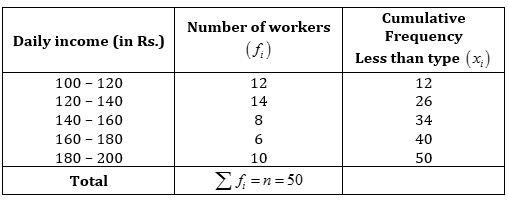Now, by drawing the points on the graph,

i.e., (120, 12); (140, 26); 160, 34); (180, 40); (200, 50)

Scale: On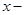axis 10 units = Rs. 10 and on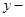axis 10 units = 5 workers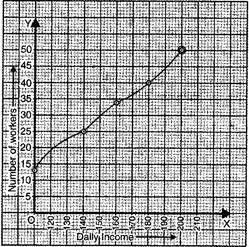NCERT Solutions for Class 10 Maths Exercise 14.4

2. During the medical checkup of 35 students of a class, their weights were recorded as follows: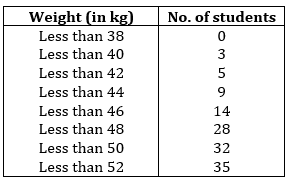Draw a less than type ogive for the given data. Hence obtain the median weight from the graph and verify the result by using the formula.

Ans.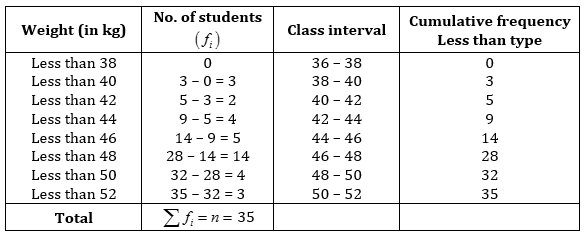Hence, the points for graph are:

(38, 0), (40, 3), (42, 5), (44, 9), (46, 14), (48, 28), (50, 32), (52, 35)

Scale: Onaxis, 10 units = 2 kg and onaxis, 10 units = 5 students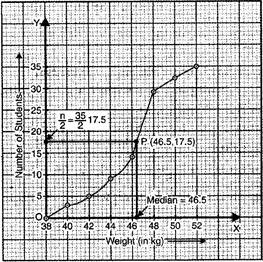From the above graph, Median = 46.5 kg, which lies in class interval 46 – 48.

Here,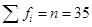, then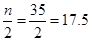, which lies in interval 46 – 48.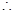Median class = 46 – 48

So,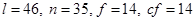and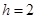Now, Median =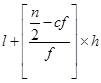=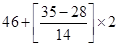=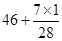= 46 + 0.5

= 46.5

Hence median weight of students is 46.5 kg.

3. The following table gives production yield per hectare of wheat of 100 farms of a village.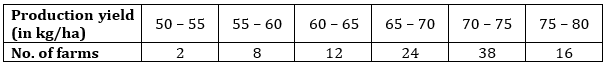Change the distribution to a more than type distribution and draw its ogive.

Ans.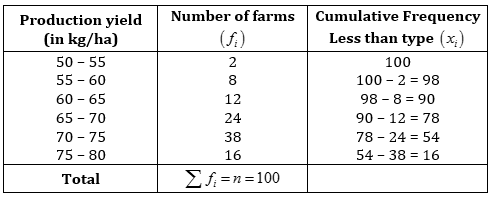The points for the graph are:

(50, 100), (55, 98), (60, 90), (65, 78), (70, 54), (75, 16)

Scale: Onaxis, 10 units = 5 kg/ha and onaxis, 10 units = 10 forms.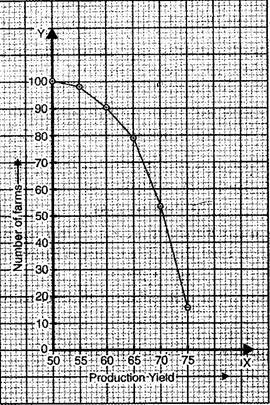## NCERT Solutions for Class 10 Maths Exercise 14.4

NCERT Solutions Class 10 Maths PDF (Download) Free from myCBSEguide app and myCBSEguide website. Ncert solution class 10 Maths includes text book solutions from Mathematics Book. NCERT Solutions for CBSE Class 10 Maths have total 15 chapters. 10 Maths NCERT Solutions in PDF for free Download on our website. Ncert Maths class 10 solutions PDF and Maths ncert class 10 PDF solutions with latest modifications and as per the latest CBSE syllabus are only available in myCBSEguide.

## CBSE app for Class 10

To download NCERT Solutions for Class 10 Maths, Computer Science, Home Science,Hindi ,English, Social Science do check myCBSEguide app or website. myCBSEguide provides sample papers with solution, test papers for chapter-wise practice, NCERT solutions, NCERT Exemplar solutions, quick revision notes for ready reference, CBSE guess papers and CBSE important question papers. Sample Paper all are made available through the best app for CBSE### 8 thoughts on “NCERT Solutions for Class 10 Maths Exercise 14.4”

1. My cbse guide is the best

2. Every thing is great but graphs are wrong. We can’t put origin’s value anything except zero.